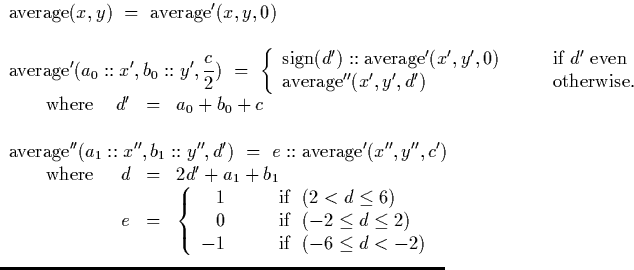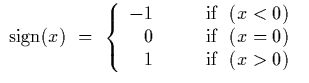Next: Analysis Up: Coding an algorithm in Previous: Signed Binary Division by

## Signed Binary Average

The implementation of the signed binary average algorithm is also extremely simple once we have the algorithm description. In section 4.3.1, we describe the algorithm as follows:The sign function is defined as follows:This is implemented in Haskell as follows.

-- sbAv : Signed binary stream average operation
sbAv :: SBinStream -> SBinStream -> SBinStream
sbAv x y = sbAv' x y 0

-- sbAv' : Auxiliary function for sbAv
sbAv' :: SBinStream -> SBinStream -> Int -> SBinStream
sbAv' (a0:x) (b0:y) c = if (d' mod 2 == 0) then
((signum d'):(sbAv' x y 0))
else sbAv'' x y d'
where d' = (a0 + b0 + 2*c)

-- sbAv'' : Auxiliary function for sbAv
sbAv'' :: SBinStream -> SBinStream -> Int -> SBinStream
sbAv'' (a1:x') (b1:y') d' = (e : sbAv' (a1:x') (b1:y') c')
where {d = (2*d' + a1 + b1);
e = if (d >  2) then  1 else
if (d < -2) then -1 else 0;
c' = d' - (2*e)}


Martin Escardo
5/11/2000